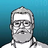V2EX = way to explore
V2EX 是一个关于分享和探索的地方

V2EX  ›  C++

# C++ RTTI 和四种类型转换

yiouejv · 2021-03-02 15:58:35 +08:00 · 1020 次点击

RTTI 是 Run Time Type Information 的缩写，从字面上来理解就是执行时期的类型信息，其重要作用就是动态判别执行时期的类型。

C++是一种静态类型语言，其数据类型是在编译期就确定的，不能在运行时更改。然而由于面向对象程序设计中多态性的要求，C++中的指针或引用(Reference)本身的类型，可能与它实际代表(指向或引用)的类型并不一致。有时我们需要将一个多态指针转换为其实际指向对象的类型，就需要知道运行时的类型信息，这就产生了运行时类型识别的要求。

``````#include<iostream>
#include<typeinfo>
using namespace std;

class Base
{
public:
virtual void funcA() { cout << "Base" << endl; }
};

class Derived : public Base
{
public:
virtual void funcB() { cout << "Derived" << endl; }
};

void funcC(Base* p)
{
Derived* dp = dynamic_cast<Derived*>(p);
if (dp != NULL) {
dp->funcB();
}
else {
p->funcA();
}
};

void funcD(Base* p)
{
Derived* dp = NULL;
if (typeid(*p) == typeid(Derived))
{
dp = static_cast<Derived*>(p);
dp->funcB();
}
else {
p->funcA();
}
}

int main(int argc, char const* argv[])
{
Base* p = new Derived;
cout << typeid(p).name() << endl;
cout << typeid(*p).name() << endl;
funcD(p);
funcC(p);
delete p;

Base* dp = new Base;
funcC(dp);
funcD(dp);
delete dp;
return 0;
}
``````

funcC 是用`dynamic_cast`类型转换是否成功来识别类型的，`dynamic_cast`操作符将基类类型对象的引用或指针转化为同一继承层次中的其他类型的引用或指针。

funcD 是用`typeid`判断基类地址是否一致的办法来识别类型的。

### typeid

`typeid`是 C++的关键字之一, 等同于`sizeof`这类 operator 。typeid 操作符的返回结果是名为 `type_info`的标准库类型的对象的引用，在头文件 typeinfo 中定义。 有两种形式:

1. typeid(type)
2. typeid(expression)

C++标准规定了其实现必须提供如下四种操作:

1. t1 == t2: 如果两个对象 t1 和 t2 类型相同，则返回 true;否则返回 false
2. t1 != t2: 如果两个对象 t1 和 t2 类型不同，则返回 true;否则返回 false
3. t.name(): 返回类型的 c-style 字符串，类型名字用系统相关的方法产生
4. t1.before(t2): 返回指出 t1 是否出现在 t2 之前 的 bool 值

`type_info`类提供了 public 虚析构函数，以使用户能够用其作为基类。它的默认构造函数和复制构造函数及赋值操作符都定义为 private，所以不能定义或复制 type_info 类型的对象。 程序中创建 type_info 对象的唯一方法是使用 typeid 操作符。 由此可见，如果把 typeid 看作函数的话，其应该是 type_info 的友元。 这具体由编译器的实现所决定，标准只要求实现为每个类型返回唯一的字符串。

RTTI 的实现原理: 通过在虚表中放一个额外的指针，每个新类只产生一个 typeinfo 实例，额外指针指向 typeinfo, typeid 返回对它的一个引用。

### static_cast

`static_cast < new_type > ( expression ) `

1. 基本数据类型之间的转换
2. initializing conversion
``````int n = static_cast<int>(3.14);
cout << "n = " << n << '\n';
vector<int> v = static_cast<vector<int>>(10);
cout << "v.size() = " << v.size() << '\n';
``````
1. 类指针或引用向下转换。
2. 将类型转为右值类型，进行 move 操作，这个在标准库中有体现（放在下一篇文章中解释）
``````vector<int> v = static_cast<vector<int>>(10);
vector<int> v2 = static_cast<vector<int>&&>(v);
cout << "after move, v.size() = " << v.size() << '\n';
cout << v.size() << endl;
``````
1. 子类数组指针向上转成父类的指针
``````struct B {
int m = 0;
void hello() const {
cout << "Hello world, this is B!\n";
}
};
struct D : B {
void hello() const {
cout << "Hello world, this is D!\n";
}
};

D a;
B* dp = static_cast<B*>(a);
dp->hello();
``````
1. 枚举转换成 int or float
``````enum E { ONE = 1, TWO, THREE };
E e = E::ONE;
int one = static_cast<int>(e);
cout << one << '\n';
``````
1. int to enum, enum to another emum
``````enum class E { ONE = 1, TWO, THREE };
enum EU { ONE = 1, TWO, THREE };

E e = E::ONE;
int one = static_cast<int>(e);
E e2 = static_cast<E>(one);
EU eu = static_cast<EU>(e2);
``````
1. void* to any type
``````int a = 100;
void* voidp = &a;
int *p = static_cast<int*>(voidp);
``````

1. static_cast 不能转换掉 expression 的 const 、volatile 和__unaligned 属性,
2. 编译器隐式执行任何类型转换都可由 static_cast 显示完成

### dynamic_cast

`dynamic_cast < new-type > ( expression )`

dynamic_cast 实现原理: 先恢复源指针的 RTTI 信息，再取目标的 RTTT 信息，比较两者是否相同，不同取目标类型的基类; 由于它需要检查一长串基类列表，故动态映射的开销比 typeid 大。

dynamic_cast 的安全性: 如实现原理所说，`dynamic_cast`会做一系列的类型检查，转换成功会返回目标类型指针，失败则会返回 NULL, 相对于`static_cast`安全，因为 `static_cast`即使转换失败也不会返回 NULL 。

``````class Base
{
public:
void func() { cout << "Base func" << endl; }
};

class Derived : public Base
{
public:
void func() { cout << "Derived func" << endl; }
};

int main(int argc, char const* argv[])
{
Derived *pd = new Derived;
pd->func();
Base* pb1 = dynamic_cast<Base*>(pd);
pb1->func();
Base* pb2 = static_cast<Base*>(pd);
pb2->func();
return 0;
}
``````

pd 指针指向的内存是子类对象，我们知道，继承子类是包含父类的，相当于在父类的基础上在添加子类的成员（如果你还不清楚的话，建议你看一下我之前的文章: 虚函数，虚表深度剖析）。 所以 pd 指针转成父类指针也是没问题的，`static_cast`也一样安全。

``````class Base
{
public:
virtual void func() { cout << "Base func" << endl; }
};

class Derived : public Base
{
public:
virtual void func() { cout << "Derived func" << endl; }
int m_value = 0;
};

int main(int argc, char const* argv[])
{
Base *pb = new Base;
pb->func();
Derived* pd1 = dynamic_cast<Derived*>(pb);
if (pd1 != NULL) {
pd1->func();
} else {
cout << "dynamic_cast failed" << endl;
}
Derived* pd2 = static_cast<Derived*>(pb);
pd2->func();
cout << "m_value: " << pd2->m_value << endl;
return 0;
}
``````

``````Base func
dynamic_cast failed
Base func  // 父类中也有这个虚函数，所以 static_cast 转换调用没出问题。
m_value: -33686019  // 这里出问题了
``````

### const_cast

`const_cast < new_type > ( expression )`

``````const int a = 26;
const int* pa = &a;
//*pa = 3;  // 编译不过，指针常量不能通过指针修改值

int* b = const_cast<int*>(pa);  // 把 const 转换掉
*b = 3;

cout << "a: " << a << endl;  // 26
cout << "*b: " << *b << endl;  // 3
``````

a 为 `const int` 类型，不可修改，pa 为 `const int*` 类型，不能通过 pa 指针修改 a 的值

b 通过 `const_cast` 转换掉了 const，成功修改了 a 的值。const_cast 的使用场景

1. 如果有一个函数，它的形参是 non-const 类型变量，而且函数不会对实参的值进行改动，这时我们可以使用类型为 const 的变量来调用函数。
``````void func(int* a)
{ }

int main()
{
const int a = 26;
const int* pa = &a;
func(const_cast<int*>(pa));
}
``````

1. 定义了一个 non-const 的变量，却使用了一个指向 const 值的指针来指向它（这不是没事找事嘛），在程序的某处我们想改变这个变量的值了，但手头只持有指针，这时`const_cast`就可以用到了
``````int a = 26;
const int* pa = &a;
// *pa = 1; 编译不过
int* pa2 = const_cast<int*>(pa);
*pa2 = 1;
``````

### reinterpret_cast

`reinterpret_cast < new_type > ( expression )`

reinterpret_ cast 通常为操作数的位模式提供较低层的重新解释。

``````int n = 9; double d = static_cast<double>(n);
cout << n << " " << d; // 输出 9 9

int n2 = 9; double d2 = reinterpret_cast<double&>(n2);
cout << n2 << " " << d2; // 输出 9 -9.25596e+61
``````reinterpret_cast 这个操作符被用于的类型转换的转换结果几乎都是未知的。

2 条回复    2021-03-03 10:22:52 +08:001 chesterzzy      2021-03-03 10:09:10 +08:00 讲得挺好的，已关注公众号2 yiouejv   OP   2021-03-03 10:22:52 +08:00 @chesterzzy 嘿嘿，一起学习